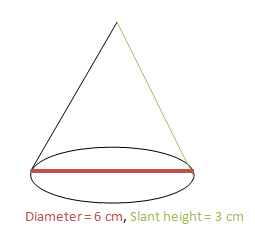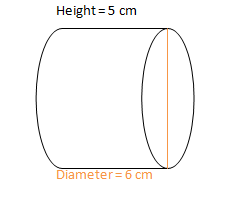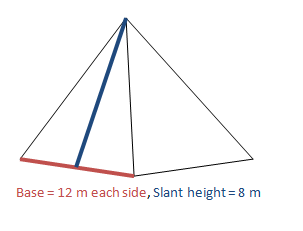# Lateral Area: Definition, Formula & Examples

Instructions:

Choose an answer and hit 'next'. You will receive your score and answers at the end.

question 1 of 3

### Find the lateral surface area of this figure.Create Your Account To Take This Quiz

As a member, you'll also get unlimited access to over 75,000 lessons in math, English, science, history, and more. Plus, get practice tests, quizzes, and personalized coaching to help you succeed.

Try it risk-free for 30 days. Cancel anytime.

### 1. Find the lateral surface area of this figure.### 2. Find the lateral surface area of this figure.Create your account to access this entire worksheet
A Premium account gives you access to all lesson, practice exams, quizzes & worksheets
Quizzes, practice exams & worksheets
Certificate of Completion
Create an account to get started

The quiz is a series of math problems. The questions will present you with figures. You will then need to find the lateral area of the given figure. Some questions will also ask you to identify the general equation for the lateral surface area of a particular type of shape.

## Quiz & Worksheet Goals

The quiz aims to improve your knowledge of these concepts:

• Lateral surface area
• Right circular cylinders
• Pyramids
• Rectangular prisms

## Skills Practiced

You will practice these skills:

• Reading comprehension - ensure that you draw the most important information from the related lateral surface area lesson
• Problem solving - use acquired knowledge to solve lateral surface area practice problems
• Interpreting information - verify that you can read information regarding lateral surface area and interpret it correctly
• Information recall - access the knowledge you've gained regarding lateral surface area

Finish the quiz and head over to the accompanying lesson. This partner lesson called Lateral Area: Definition, Formula & Examples will help you explore the nuances of lateral surface area and its various equations for different shapes. The lesson covers these objectives:

• Defining the term lateral
• Exploring the idea of lateral surface area
• Relating lateral surface area to various shapes
• Walking through example lateral surface area problems
Final ExamGMAT Prep: Tutoring Solution
Status: Not Started
Chapter ExamPerimeter, Area & Volume: Tutoring Solution
Status: Not Started

Support